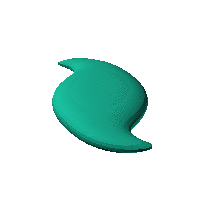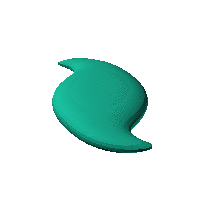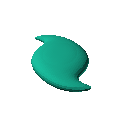Blob Farm# blob05The blob in all its glory:Cute little twist. Has possibilites as a case.

$r\sqrt{{x}^{2}+{y}^{2}}$
$-2.3254629358757537\theta +0.05\text{}\left({r}^{2}+r\right)$Click on the snapshot to download the blob's stl file.Octave Code:
```1; # Prevent Octave from thinking that this is a function
# though one is defined here

function w = f(x2,y2,z2,c,r,e)
x  = (x2-c(1))/r(1);
y  = (y2-c(2))/r(2);
z  = (z2-c(3))/r(3);
# function at origin must be <0, and >0 far enough away.  w=0 defines the surface
th = atan2(x,y);
r = sqrt(x.^2+y.^2);
phi = th + (r+r.^2)*0.05;
w = (1.0)./(abs(0.2*cos(phi).*r).^2+abs(0.8*sin(phi).*r).^2.5+abs(2*z).^2.5)+(0.6)./(abs(r*0.3).^2.5+abs(z).^2.5)-2.0;

endfunction;
```
GNU Octave